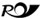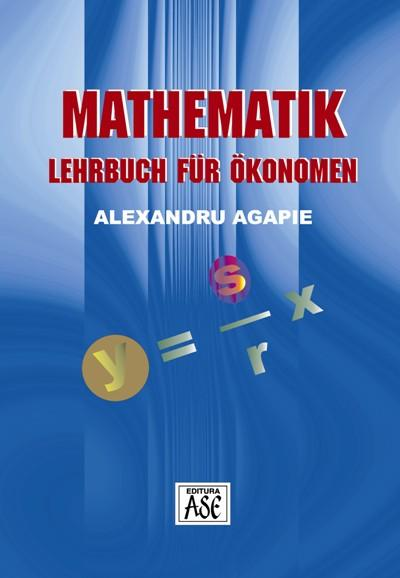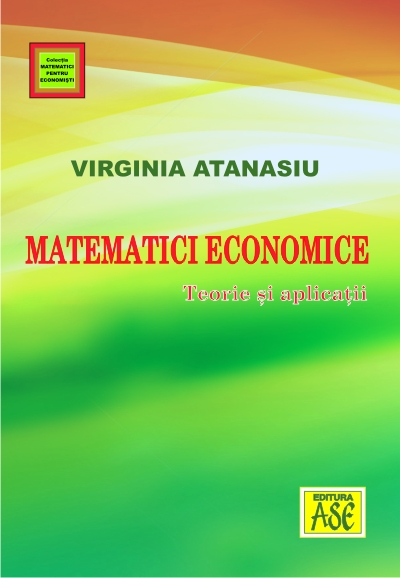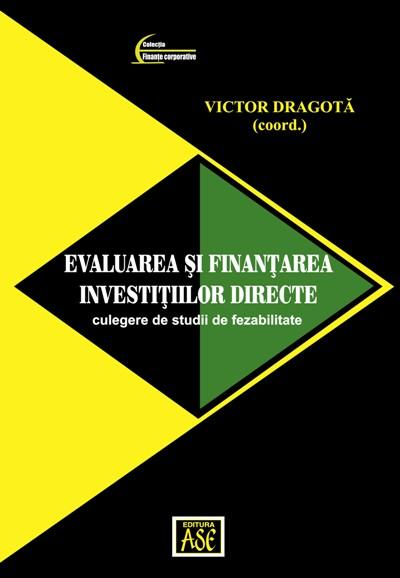# Elements of graph theory, game theory and mathematical statistics. Mathematical models in economics. Theory and Applications

Autors: Virginia Atanasiu

Year of appearance: 2015

ISBN: 978-606-505-865-1

53,00 lei In stock: YESRomanian Post delivery

This study examines various theoretical and practical problems in the fields of graph theory, game theory and mathematical statistics, probability theory and mathematical statistics, in order to provide a mathematical basis necessary and useful at the same time, for the decisions specific economic and financial business management.

The book contains many theoretical results a specific character for the economic activities and also includes many formulas, procedures and practical mathematical models, that may occur in the diversity of business and which are illustrated later by several tens of numerical examples solved.

Like any product of teaching and research of long term, the work is a synthesis of preoccupations, ideas and theoretical and practical results, where the mathematical calculation proves its usefulness.

## Similar products### Mathematics. Textbooks for economics

19,00 lei### Matematici economice. Teorie si aplicatii

70,00 lei### Mathematical optimization methods with applications in economy

22,90 lei40,40 lei

## Others have bought49,50 lei